1800-1023-196

+91-120-4616500

# Factors Affecting the Electrolytic Conductance

## Factors Affecting the Electrolytic Conductance

In general, conductance of an electrolyte depends upon the following factors,

(1) Nature of electrolyte: The conductance of an electrolyte depends upon the number of ions present in the solution. Therefore, the greater the number of ions in the solution the greater is the conductance. The number of ions produced by an electrolyte depends upon its nature. The strong electrolytes dissociate almost completely into ions in solutions and, therefore, their solutions have high conductance. On the other hand, weak electrolytes, dissociate to only small extents and give lesser number of ions. Therefore, the solutions of weak electrolytes have low conductance.

(2) Concentration of the solution: The molar conductance of electrolytic solution varies with the concentration of the electrolyte. In general, the molar conductance of an electrolyte increases with decrease in concentration or increase in dilution.

The molar conductance of strong electrolyte (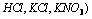as well as weak electrolytes (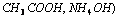increase with decrease in concentration or increase in dilution. The variation is however different for strong and weak electrolytes.

The variation of molar conductance with concentration can be explained on the basis of conducting ability of ions for weak and strong electrolytes.

For weak electrolytes the variation of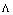with dilution can be explained on the bases of number of ions in solution. The number of ions furnished by an electrolyte in solution depends upon the degree of dissociation with dilution. With the increase in dilution, the degree of dissociation increases and as a result molar conductance increases. The limiting value of molar conductance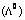corresponds to degree of dissociation equal to 1 i.e., the whole of the electrolyte dissociates.

Thus, the degree of dissociation can be calculated at any concentration as,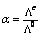where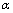is the degree of dissociation,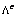is the molar conductance at concentration C and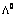is the molar conductance at infinite dilution.

For strong electrolytes, there is no increase in the number of ions with dilution because strong electrolytes are completely ionised in solution at all concentrations (By definition). However, in concentrated solutions of strong electrolytes there are strong forces of attraction between the ions of opposite charges called inter-ionic forces. Due to these inter-ionic forces the conducting ability of the ions is less in concentrated solutions. With dilution, the ions become far apart from one another and inter-ionic forces decrease. As a result, molar conductivity increases with dilution. When the concentration of the solution becomes very-very low, the inter-ionic attractions become negligible and the molar conductance approaches the limiting value called molar conductance at infinite dilution. This value is characteristic of each electrolyte.

(3) Temperature: The conductivity of an electrolyte depends upon the temperature. With increase in temperature, the conductivity of an electrolyte increases.

Migration of ions

Electricity is carried out through the solution of an electrolyte by migration of ions. Therefore,

(1) Ions move toward oppositely charged electrodes at different speeds.

(2) During electrolysis, ions are discharged or liberated in equivalent amounts at the two electrodes, no matter what their relative speed is.

(3) Concentration of the electrolyte changes around the electrode due to difference in the speed of the ions.

(4) Loss of concentration around any electrode is proportional to the speed of the ion that moves away from the electrode, so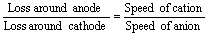The relation is valid only when the discharged ions do not react with atoms of the electrodes. But when the ions combine with the material of the electrode, the concentration around the electrode shows an increase.

## NEET & AIIMS Exam Sample Papers

 AIIMS SAMPLE PAPERS View More NEET SAMPLE PAPERS View More